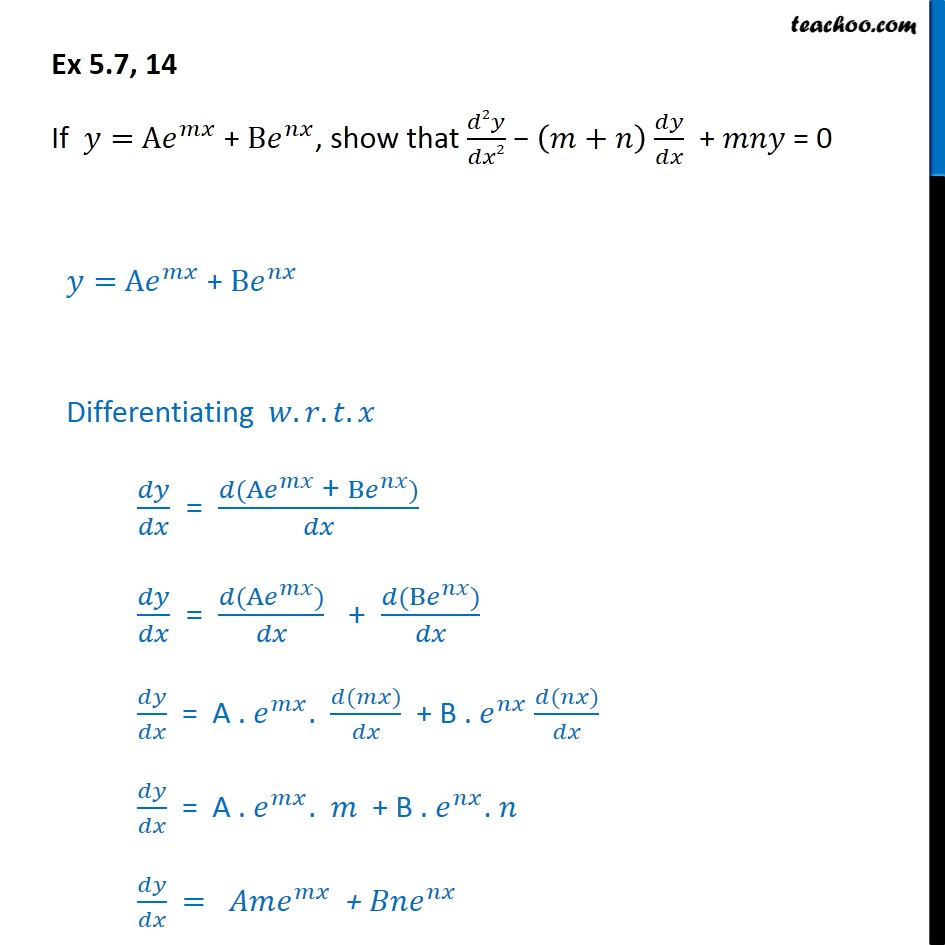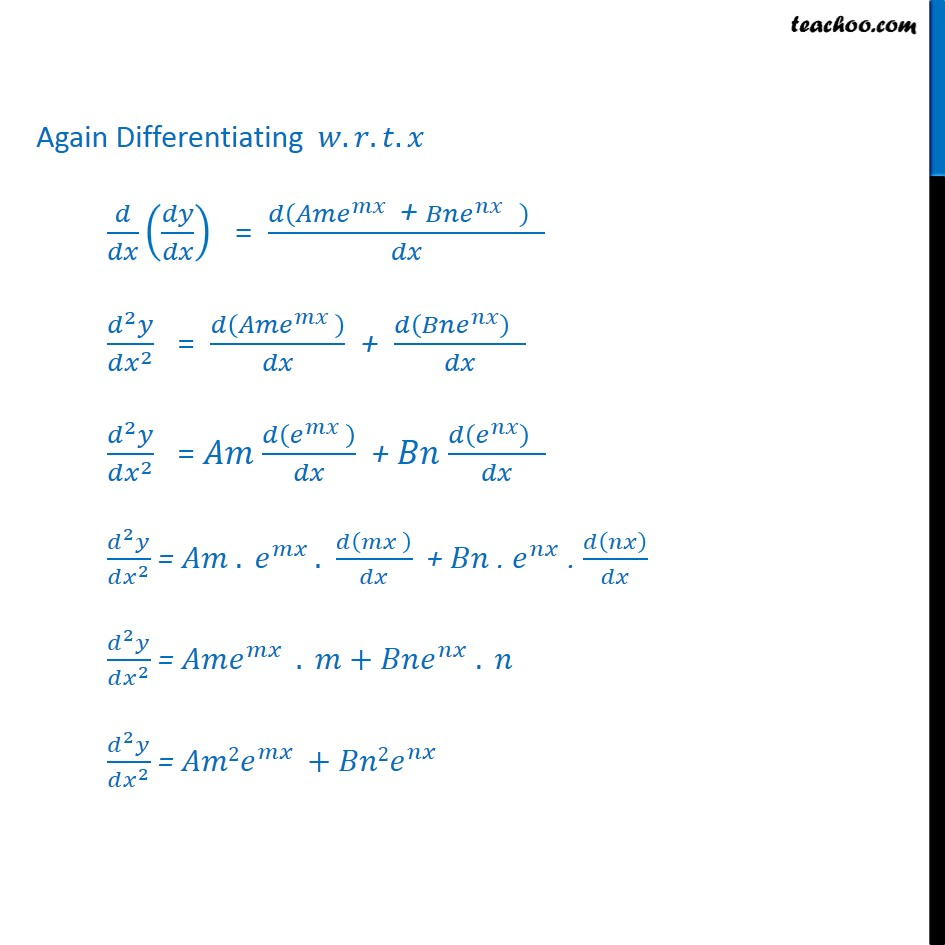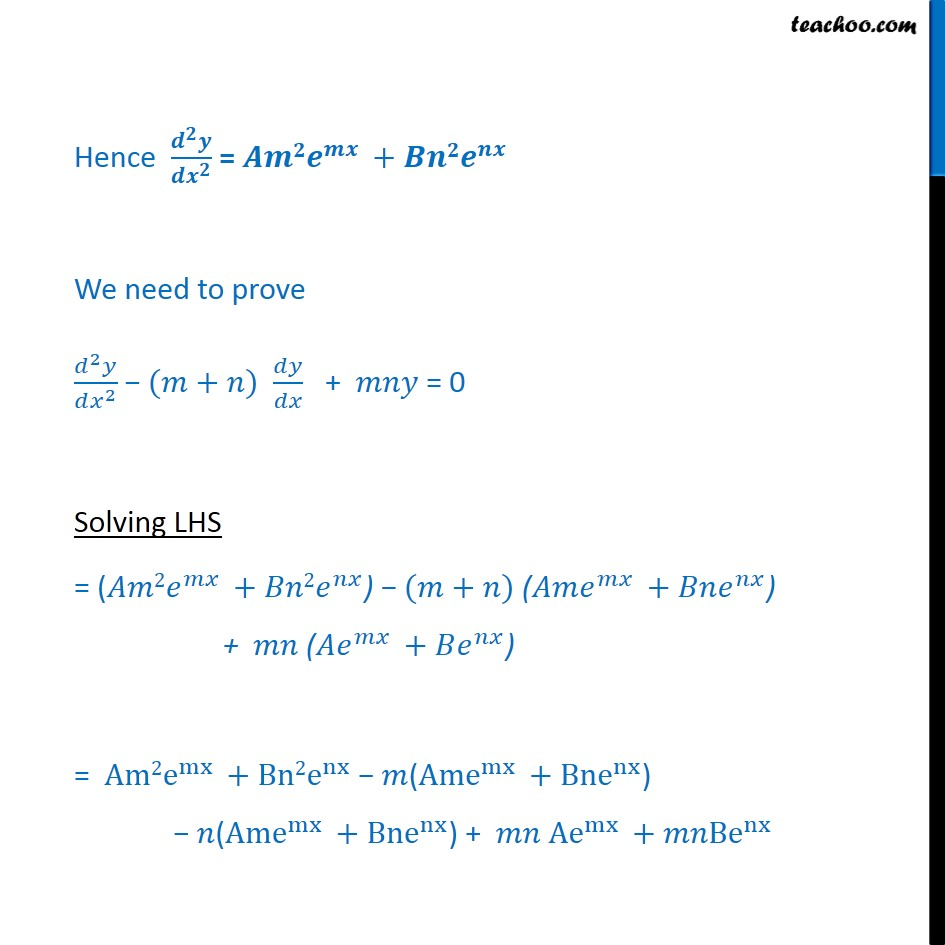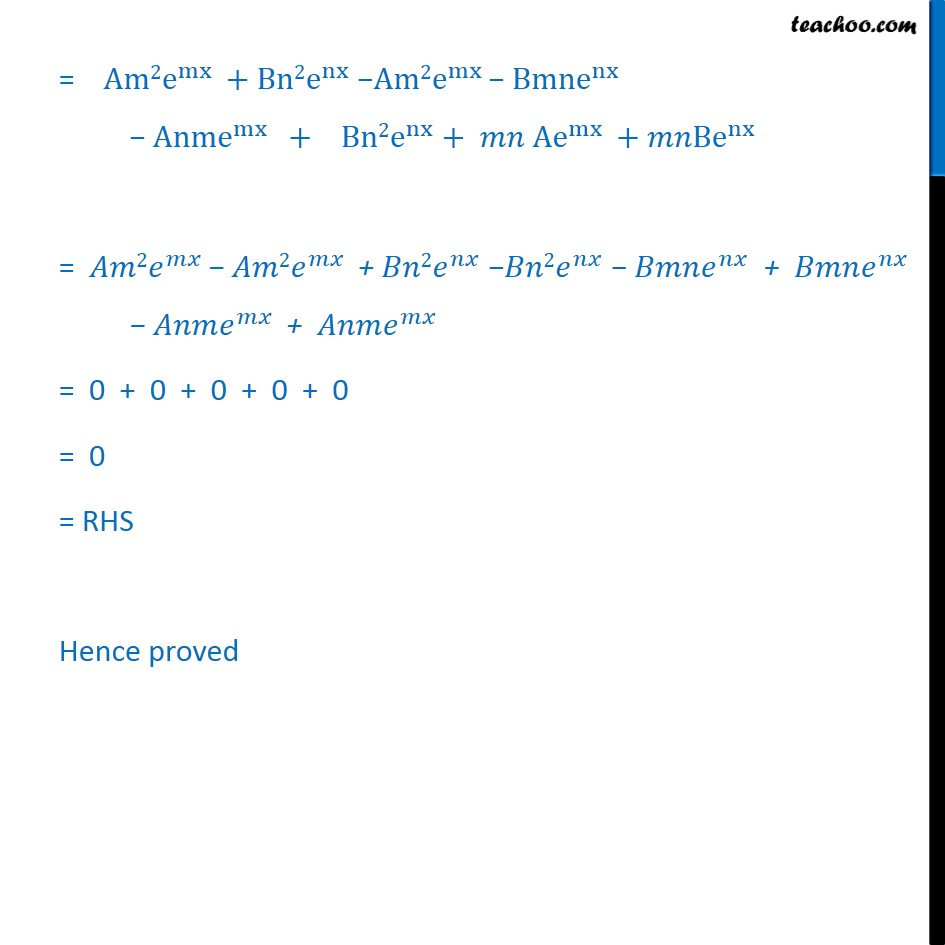1. Chapter 5 Class 12 Continuity and Differentiability
2. Serial order wise
3. Ex 5.7

Transcript

Ex 5.7, 14 If 𝑦= A𝑒﷮𝑚𝑥﷯ + B𝑒﷮𝑛𝑥﷯, show that 𝑑2𝑦﷮𝑑𝑥2﷯ − 𝑚+𝑛﷯ 𝑑𝑦﷮𝑑𝑥﷯ + 𝑚𝑛𝑦 = 0 𝑦= A𝑒﷮𝑚𝑥﷯ + B𝑒﷮𝑛𝑥﷯ Differentiating 𝑤.𝑟.𝑡.𝑥 𝑑𝑦﷮𝑑𝑥﷯ = 𝑑( A𝑒﷮𝑚𝑥﷯ + B𝑒﷮𝑛𝑥﷯)﷮𝑑𝑥﷯ 𝑑𝑦﷮𝑑𝑥﷯ = 𝑑( A𝑒﷮𝑚𝑥﷯)﷮𝑑𝑥﷯ + 𝑑( B𝑒﷮𝑛𝑥﷯)﷮𝑑𝑥﷯ 𝑑𝑦﷮𝑑𝑥﷯ = A . 𝑒﷮𝑚𝑥﷯. 𝑑(𝑚𝑥)﷮𝑑𝑥﷯ + B . 𝑒﷮𝑛𝑥﷯ 𝑑(𝑛𝑥)﷮𝑑𝑥﷯ 𝑑𝑦﷮𝑑𝑥﷯ = A . 𝑒﷮𝑚𝑥﷯. 𝑚 + B . 𝑒﷮𝑛𝑥﷯. 𝑛 𝑑𝑦﷮𝑑𝑥﷯ = 𝐴𝑚 𝑒﷮𝑚𝑥﷯ + 𝐵𝑛 𝑒﷮𝑛𝑥﷯ Again Differentiating 𝑤.𝑟.𝑡.𝑥 𝑑﷮𝑑𝑥﷯ 𝑑𝑦﷮𝑑𝑥﷯﷯ = 𝑑 𝐴𝑚 𝑒﷮𝑚𝑥﷯ + 𝐵𝑛 𝑒﷮𝑛𝑥﷯ ﷯ ﷮𝑑𝑥﷯ 𝑑﷮2﷯𝑦﷮𝑑 𝑥﷮2﷯﷯ = 𝑑 𝐴𝑚 𝑒﷮𝑚𝑥﷯ ﷯﷮𝑑𝑥﷯ + 𝑑 𝐵𝑛 𝑒﷮𝑛𝑥﷯﷯ ﷮𝑑𝑥﷯ 𝑑﷮2﷯𝑦﷮𝑑 𝑥﷮2﷯﷯ = 𝐴𝑚 𝑑 𝑒﷮𝑚𝑥﷯ ﷯﷮𝑑𝑥﷯ + 𝐵𝑛 𝑑 𝑒﷮𝑛𝑥﷯﷯ ﷮𝑑𝑥﷯ 𝑑﷮2﷯𝑦﷮𝑑 𝑥﷮2﷯﷯ = 𝐴𝑚 . 𝑒﷮𝑚𝑥 ﷯. 𝑑 𝑚𝑥 ﷯﷮𝑑𝑥﷯ + 𝐵𝑛 . 𝑒﷮𝑛𝑥﷯ . 𝑑 𝑛𝑥﷯﷮𝑑𝑥﷯ 𝑑﷮2﷯𝑦﷮𝑑 𝑥﷮2﷯﷯ = 𝐴𝑚 𝑒﷮𝑚𝑥 ﷯ . 𝑚+𝐵𝑛 𝑒﷮𝑛𝑥﷯ . 𝑛 𝑑﷮2﷯𝑦﷮𝑑 𝑥﷮2﷯﷯ = 𝐴𝑚2 𝑒﷮𝑚𝑥 ﷯+𝐵𝑛2 𝑒﷮𝑛𝑥﷯ Hence 𝒅﷮𝟐﷯𝒚﷮𝒅 𝒙﷮𝟐﷯﷯ = 𝑨𝒎𝟐 𝒆﷮𝒎𝒙 ﷯+𝑩𝒏𝟐 𝒆﷮𝒏𝒙﷯ We need to prove 𝑑﷮2﷯𝑦﷮𝑑 𝑥﷮2﷯﷯ − (𝑚+𝑛) 𝑑𝑦﷮𝑑𝑥﷯ + 𝑚𝑛𝑦 = 0 Solving LHS = (𝐴𝑚2 𝑒﷮𝑚𝑥 ﷯+𝐵𝑛2 𝑒﷮𝑛𝑥﷯) − (𝑚+𝑛) (𝐴𝑚 𝑒﷮𝑚𝑥 ﷯+𝐵𝑛 𝑒﷮𝑛𝑥﷯) + 𝑚𝑛 (𝐴 𝑒﷮𝑚𝑥 ﷯+𝐵 𝑒﷮𝑛𝑥﷯) = Am2 e﷮mx ﷯+Bn2 e﷮nx﷯ − 𝑚(Am e﷮mx ﷯+Bn e﷮nx﷯) − 𝑛(Am e﷮mx ﷯+Bn e﷮nx﷯) + 𝑚𝑛 A e﷮mx ﷯+𝑚𝑛B e﷮nx﷯ = Am2 e﷮mx ﷯+Bn2 e﷮nx﷯ −Am2 e﷮mx ﷯− Bmn e﷮nx﷯ − Anm e﷮mx ﷯ + Bn2 e﷮nx﷯+ 𝑚𝑛 A e﷮mx ﷯+𝑚𝑛B e﷮nx﷯ = 𝐴𝑚2 𝑒﷮𝑚𝑥 ﷯− 𝐴𝑚2 𝑒﷮𝑚𝑥 ﷯ + 𝐵𝑛2 𝑒﷮𝑛𝑥﷯ −𝐵𝑛2 𝑒﷮𝑛𝑥﷯ − 𝐵𝑚𝑛 𝑒﷮𝑛𝑥﷯ + 𝐵𝑚𝑛 𝑒﷮𝑛𝑥﷯ − 𝐴𝑛𝑚 𝑒﷮𝑚𝑥 ﷯ + 𝐴𝑛𝑚 𝑒﷮𝑚𝑥 ﷯ = 0 + 0 + 0 + 0 + 0 = 0 = RHS Hence proved

Ex 5.7